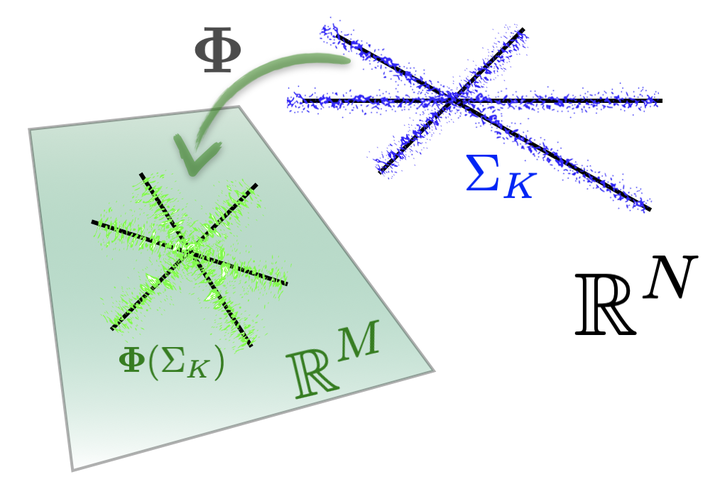# New class of RIP matrices?Wow, almost one year and half without any post here… Shame on me! I’ll try to be more productive with shorter posts now ;-)

I just found this interesting paper about concentration properties of submodular function (very common in “Graph Cut” methods for instance) on arxiv:

A note on concentration of submodular functions. (arXiv:1005.2791v1 [cs.DM])

Jan Vondrak, May 18, 2010

We survey a few concentration inequalities for submodular and fractionally subadditive functions of independent random variables, implied by the entropy method for self-bounding functions. The power of these concentration bounds is that they are dimension-free, in particular implying standard deviation $$O(\sqrt{\mathbb E[f]})$$ rather than $$O(\sqrt{n})$$ which can be obtained for any 1-Lipschitz function of n variables.

In particular, the author shows some interesting concentration results in his Corollary 3.2:

Without having performed any developments, I’m wondering if this result could serve to define a new class of matrices (or non-linear operators) satisfying either the Johnson-Lindenstrauss Lemma or the Restricted Isometry Property.

For instance, by starting from Bernoulli vectors $$X$$, i.e., the rows of a sensing matrix, and defining some specific submodular (or self-bounding) functions $$f$$ (e.g., $$f(X_1,\cdots, X_n) = g(X^T a)$$ for some sparse vector $$a$$ and a “kind” function $$g$$), I’m wondering if the concentration results above are better than those coming from the classical concentration inequalities (based on the Lipschitz properties of $$f$$ or $$g$$. See e.g., the books of Ledoux and Talagrand)?

Ok, all this is perhaps just too hasty thoughts …. written before a mug of black coffee ;-)